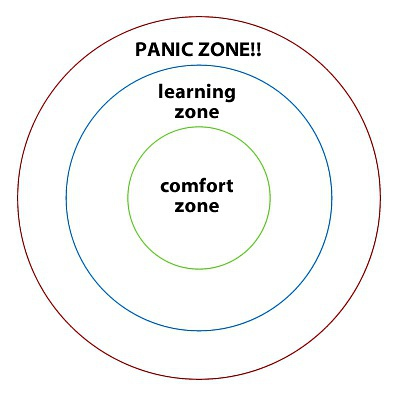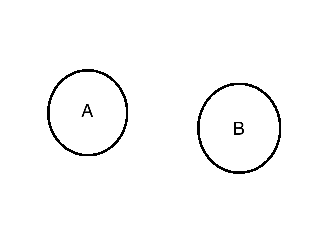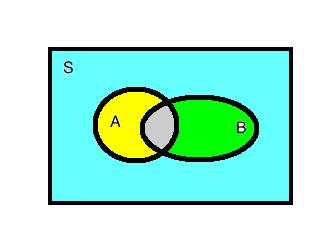Abstract: 本文介绍事件的独立，以及派生出来的多事件独立，以及条件独立
Keywords: Independent Events，Independent of Several Events，Coditionally Independent Events

# 独立事件## 独立事件 Independent Events

$$Pr(A|B)=\frac{Pr(A\cap B)}{Pr(B)}\quad where \quad Pr(B)>0\\ Pr(A\cap B)=Pr(A|B)Pr(B) \quad where \quad Pr(B)>0$$

$$Pr(A\cap B)=Pr(A)Pr(B)$$

### 两个事件独立 Independence of Two Events

Definition Independent Events.Two events A and B are independent if
$$Pr(A\cap B)=Pr(A)Pr(B)$$

### 互斥事件和独立事件 Exclusive Events and independent Events### 补集之间的独立 Independence of Complements

Theorem If two events A and B are independent,then the events $A$ and $B^c$ are also independent.

$$A=(A\cap B^c)\cup(A\cap B)\\ Pr(A)=Pr(A\cap B^c)+Pr(A\cap B)\\ Pr(A\cap B)=Pr(A)Pr(B)\\ Pr(A\cap B^c)=Pr(A)-Pr(A)Pr(B)=Pr(A)(1-Pr(B))=Pr(A)Pr(B^c)$$
Q.E.D

## 多事件独立 Independent of Several Events

Definition Mutually Independent Events The k events $A_1,\dots,A_k$ are independent or mutually independent if for every subset $A_{i_1},\dots,A_{i_j}$ of j of these events($j=2,3,\dots,k$)
$$Pr(A_{i_1}\cap \dots \cap A_{i_j} )=Pr(A_{i_1})\dots Pr(A_{i_j})$$

$$Pr(A\cap B)=Pr(A)Pr(B)\\ Pr(A\cap C)=Pr(A)Pr(C)\\ Pr(B\cap C)=Pr(B)Pr(C)\\ Pr(A\cap B\cap C)=Pr(A)Pr(B)Pr(C)$$

1. $Pr(A\cap B\cap C)=Pr(A)Pr(B)Pr(C)$ 不能保证三个事件独立
2. 这三个也不行
$$Pr(A\cap B)=Pr(A)Pr(B)\\ Pr(A\cap C)=Pr(A)Pr(C)\\ Pr(B\cap C)=Pr(B)Pr(C)\\$$

①$A={HH,HT}$

②$B={HH,TH}$

③$C={HH,TT}$

$$Pr(A\cap B)=Pr(B\cap C)=Pr(A\cap C)=Pr(A\cap B \cap C)=\frac{1}{4}$$

$$Pr(A\cap B)=Pr(A)Pr(B)\\ Pr(A\cap C)=Pr(A)Pr(C)\\ Pr(B\cap C)=Pr(B)Pr(C)\\$$

### 独立和条件概率 Independence and Conditional Probability

Theorem Let $A_1,A_2,\dots,A_k$ be events such that $Pr(A_1\cap \dots \cap A_k)>0$ .Then $A_1\dots A_m$ and $A_1\dots A_l$ of$A_1\dots A_k$ we have
$$Pr(A_{i_1}\cap \dots \cap A_{i_m}|A_{j_1}\cap \dots \cap A_{j_l})=Pr(A_{i_1}\cap \dots \cap A_{i_m})$$

## 条件独立事件 Coditionally Independent Events

Theorem Conditional Independence We say that event $A_1\dots A_k$ are conditional independent given B if,for every subcollection $A_{i_1},\dots ,A_{i_j}$ of $j$ of these events ($j=2,3,\dots,k$)
$$Pr(A_{i_1}\cap \dots \cap A_{i_j}|B)=Pr(A_{i_1}|B)\dots Pr(A_{i_j}|B)$$

Theorem Suppose that $A_1,A_2$ and $B$ are events such that $Pr(A_1\cap B)>0$ Then $A_1$ and $A_2$ are conditional independent given $B$ if and only if $Pr(A_2|A_1\cap B)=Pr(A_2|B)$

$$Pr(A_1\cap A_2 \cap B)=Pr(A_1\cap A_2|B)Pr(B)=Pr(A_2|A_1\cap B)Pr(A_1|B)Pr(B)\\ then: \quad Pr(A_1\cap A_2|B)=Pr(A_2|A_1\cap B)Pr(A_1|B)\\ for: \quad Pr(A_1\cap A_2|B)=Pr(A_1|B)Pr(A_2|B)\\ so: \quad Pr(A_1|B)Pr(A_2|B)=Pr(A_2|A_1\cap B)Pr(A_1|B)\\ Pr(A_2|A_1\cap B)=Pr(A_2|B)$$

0%